# Written in Stone: The World’s First Trigonometry Revealed in an Ancient Babylonian Tablet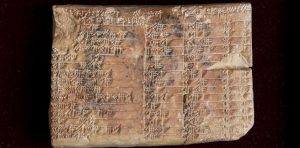The Plimpton 322 tablet. UNSW/Andrew Kelly, Creative Commons

A 3,700-year old Babylonian clay tablet reveals an ancient method of constructing right-angled triangles that makes it the world’s oldest and most accurate trigonometric table.

By Dr. Daniel Mansfield (left) and Dr. Norman Wilderberger (right) / 08.24.2017
Mansfield: Associate Lecturer in Mathematics
Wilderberger: Associate Professor in Mathematics
University of New South Wales

The ancient Babylonians – who lived from about 4,000BCE in what is now Iraq – had a long forgotten understanding of right-angled triangles that was much simpler and more accurate than the conventional trigonometry we are taught in schools.

Our new research, published in Historia Mathematica, shows that the Babylonians were able to construct a trigonometric table using only the exact ratios of sides of a right-angled triangle. This is a completely different form of trigonometry that does not need the familiar modern concept of angles.

At school we are told that the shape of a right-angled triangle depends upon the other two angles. The angle is related to the circumference of a circle, which is divided into 360 parts or degrees. This angle is then used to describe the ratios of the sides of the right-angled triangle through sin, cos and tan.

But circles and right-angled triangles are very different, and the price of having simple values for the angle is borne by the ratios, which are very complicated and must be approximated.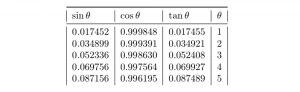The three ratios of a modern trigonometric table, rounded to six decimal places, with auxiliary angle Θ in degrees. Daniel MansfieldAuthor provided

This approach can be traced back to the Greek astronomer and mathematician Hipparchus of Nicaea (who died after 127 BCE). He is said to be the father of trigonometry because he used his table of chords to calculate orbits of the Moon and Sun.

But our new research shows this was not the first, or only, or best approach to trigonometry.

## Babylonian trigonometry

The Babylonians discovered their own unique form of trigonometry during the Old Babylonian period (1900-1600BCE), more than 1,500 years earlier than the Greek form.

Remarkably, their trigonometry contains none of the hallmarks of our modern trigonometry – it does not use angles and it does not use approximation.

The Babylonians had a completely different conceptualisation of a right triangle. They saw it as half of a rectangle, and due to their sophisticated sexagesimal (base 60) number system they were able to construct a wide variety of right triangles using only exact ratios.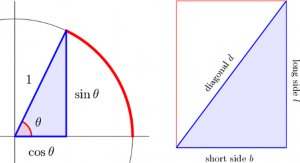The Greek (left) and Babylonian (right) conceptualisation of a right triangle. Notably the Babylonians did not use angles to describe a right triangle. Daniel MansfieldAuthor provided

The sexagesimal system is better suited for exact calculation. For example, if you divide one hour by three then you get exactly 20 minutes. But if you divide one dollar by three then you get 33 cents, with 1 cent left over. The fundamental difference is the convention to treat hours and dollars in different number systems: time is sexagesimal and dollars are decimal.

The Babylonians knew that their sexagesmial number system allowed for many more exact divisions. For a more sophisticated example, 1 hour divided by 48 is 1 minute and 15 seconds.

This precise arithmetic of the Babylonians also influenced their geometry, which they preferred to be exact. They were able to generate a wide variety of right-angled triangles within exact ratios b/l and d/l, where b, l and d are the short side, long side and diagonal of a rectangle.

The ratio b/l was particularly important to the ancient Babylonians and Egyptians because they used this ratio to measure steepness.

## The Plimpton 322 tablet

We now know that the Babylonians studied trigonometry because we have a fragment of a one of their trigonometric tables.

Plimpton 322 is a broken clay tablet from the ancient city of Larsa, which was located near Tell as-Senkereh in modern day Iraq. The tablet was written between 1822-1762BCE.

In the 1920s the archaeologist, academic and adventurer Edgar J Banks sold the tablet to the American publisher and philanthropist George Arthur Plimpton.

Plimpton bequeathed his entire collection of mathematical artefacts to Columbia University in 1936, and it resides there today in the Rare Book and Manuscript Library. It’s available online through the Cuneiform Digital Library Initiative.

In 1945 the tablet was revealed to contain a highly sophisticated sequence of integer numbers that satisfy the Pythagorean equation a2+b2=c2, known as Pythagorean triples.

This is the fundamental relationship of the three sides of a right-angled triangle, and this discovery proved that the Babylonians knew this relationship more than 1,000 years before the Greek mathematician Pythagoras was born.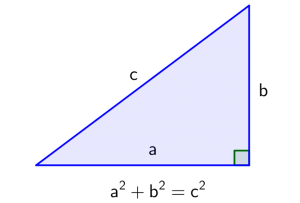The fundamental relation between the side lengths of a right triangle. In modern times this is called Pythagoras’ theorem, but it was known to the Babylonians more than 1,000 years before Pythagoras was born.

Plimpton 322 has ruled space on the reverse which indicates that additional rows were intended. In 1964, the Yale based science historian Derek J de Solla Price discovered the pattern behind the complex sequence of Pythagorean triples and we now know that it was originally intended to contain 38 rows in total.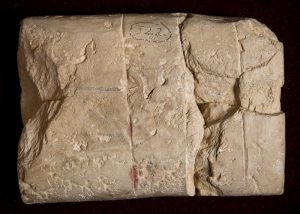The other side of the Plimpton 322 tablet. UNSW/Andrew KellyAuthor provided

The tablet also has missing columns, and in 1981 the Swedish mathematics historian Jöran Friberg conjectured that the missing columns should be the ratios b/l and d/l. But the tablet’s purpose remained elusive.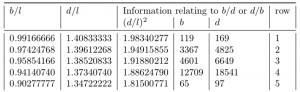The first five rows of Plimpton 322, with reconstructed columns and numbers written in decimal.

The surviving fragment of Plimpton 322 starts with the Pythagorean triple 119, 120, 169. The next triple is 3367, 3456, 4825. This makes sense when you realise that the first triple is almost a square (which is an extreme kind of rectangle), and the next is slightly flatter. In fact the right-angled triangles are slowly but steadily getting flatter throughout the entire sequence.

Watch the triangles change shape as we go down the list

So the trigonometric nature of this table is suggested by the information on the surviving fragment alone, but it is even more apparent from the reconstructed tablet.

This argument must be made carefully because modern notions such as angle were not present at the time Plimpton 322 was written. How then might it be a trigonometric table?

Fundamentally a trigonometric table must describe three ratios of a right triangle. So we throw away sin and cos and instead start with the ratios b/l and d/l. The ratio which replaces tan would then be b/d or d/b, but neither can be expressed exactly in sexagesimal.

Instead, information about this ratio is split into three columns of exact numbers. A squared index and simplified values of b and d to help the scribe make their own approximation to b/d or d/b.

## No approximation

The most remarkable aspect of Babylonian trigonometry is its precision. Babylonian trigonometry is exact, whereas we are accustomed to approximate trigonometry.

The Babylonian approach is also much simpler because it only uses exact ratios. There are no irrational numbers and no angles, and this means that there is also no sin, cos or tan or approximation.

It is difficult to say why this approach to trigonometry has not survived. Perhaps it went out of fashion because the Greek approach using angles is more suitable for astronomical calculations. Perhaps this understanding was lost in 1762BCE when Larsa was captured by Hammurabi of Babylon. Without evidence, we can only speculate.

We are only beginning to understand this ancient civilisation, which is likely to hold many more secrets waiting to be discovered.

Originally published by The Conversation under a Creative Commons Attribution/No derivatives license.

comments